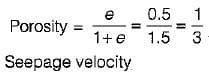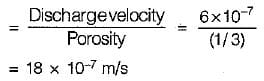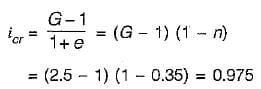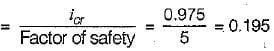# Test: Seepage Analysis - 2

## 8 Questions MCQ Test GATE Civil Engineering (CE) 2023 Mock Test Series | Test: Seepage Analysis - 2

Description
Attempt Test: Seepage Analysis - 2 | 8 questions in 25 minutes | Mock test for Civil Engineering (CE) preparation | Free important questions MCQ to study GATE Civil Engineering (CE) 2023 Mock Test Series for Civil Engineering (CE) Exam | Download free PDF with solutions
QUESTION: 1

### Darcy’s law for flow through soil is valid if Reynold's number is less than

Solution:

Darcy's law is valid if the flow through soil is laminar and Reynolds number is less than 1.

QUESTION: 2

### Consider the following statements: 1. Seepage force is applied by flowing water to the soil skeleton through frictional drag. 2. The magnitude of seepage force per unit volume of soil at any point is equal to γw/i, where γw is the unit weight of water and i is the hydraulic gradient at that point. 3. In a soil mass subjected to upward flow of water, quick sand condition develops when pore pressure is equal to the total stress Which of these statements is/are correct?

Solution:

Seepage force is due to frictional drag and its magnitude is given by iγw per unit volume of soil. For quick condition during upward flow.
Effective stress = total stress - pore pressure So statement 1 and 3 is correct.

*Multiple options can be correct
QUESTION: 3

### Which one of the following explains the flow condition occurring within a soil when its effective pressure is reduced to zero?

Solution:

When the seepage of water is upward in a soil layer so much that seepage force decreases the inter particle forces and effective stress is reduced to zero, quick sand condition occurs. Liquefaction of sand is also a phenomenon when effective stress of soil is reduced to zero, it usually occurs when sand deposit is shaken due to an earthquake or any other oscillatory load which increases the pore water pressure.

QUESTION: 4

A soil has a discharge velocity of 6 x 10-7 m/s and a void ratio of 0.5. Its seepage velocity is

Solution:QUESTION: 5

For an anisotropic soil, permeabilities in x and y directions are Kx and Ky respectively in a two dimensional flow. The effective permeability Keq for the soil is given by

Solution:
QUESTION: 6

To provide safety against piping failure, with a factor of safety of 5, what should be the maximum permissible exit gradient for soil with specific gravity of 2.5 and porosity of 0.35?

Solution:QUESTION: 7

During seepage through an earth mass, the direction of seepage is

Solution:
QUESTION: 8

The upstream slope of an earth dam under steady seepage condition is

Solution:Use Code STAYHOME200 and get INR 200 additional OFF Use Coupon Code